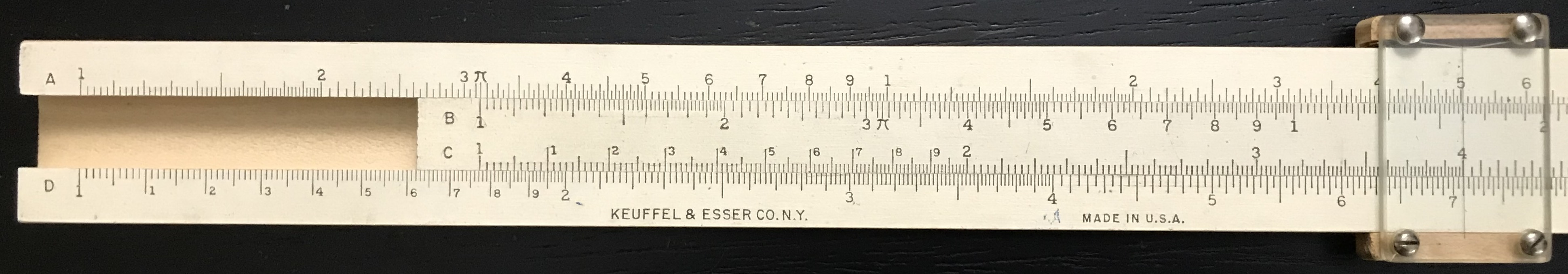## 3.3 Gauge Marks

Many slide rules have additional markings on their scales called “Gauge Marks” (sometimes called gauge points). A gauge mark indicates a commonly used numerical value that can be found quickly for use in certain computations. The most common mark is usually for the value of $$\pi$$ = 3.14159… On the rule used in the examples above, we see a $$\pi$$ symbol on the A and B scales. Consider its use for the following (which is similar in technique to our last example): Set the 1 on the B scale to the $$\pi$$ gauge mark on the A scale. On the D scale directly below the $$\pi$$ will be $$\sqrt{\pi} \approx 1.77$$. Now look at numbers along the C scale; take 4, for instance. The number below it on the D scale will be $$\sqrt{\pi} \times 4 \approx$$ 7.09. Looking directly up on the A scale we see the “square” of this result, which will be $$\pi \times 4^2 = 7.09^2 \approx$$ 50.3. That is, with the B scale gauged to $$\pi$$ on the A scale, the A scale will give the area of a circle for any radius read off from the C scale. Check: Suppose the radius is 2; then without moving the slide at all one can find the value of 2 on the C scale and directly read off the area $$\pi\times 2^2$$ = 12.5 on the A scale right above. A great time saver when repeating many similar calculations!Using a $$\pi$$ Gauge Mark.

More sophisticated slide rules might have several gauge marks on them, such as for $$\sqrt{\pi}$$ = 1.772…, $$\sqrt{\pi/4}$$ = 0.886…, $$e$$ = 2.718…, Watts/Horsepower = 746 (or, 7.46 on the rule), degrees/radian = 57.30… (or, 5.73 on the rule), and many others.